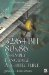Chapter 12: Floating-Point Vector Addition and SubtractionOverview

The topic of floating-point was discussed back in Chapter 8, "Floating-Point Anyone?" As the same methodologies of SIMD processing learned in Chapter 7, "Integer Math," apply for packed floating-point, it does not matter whether one is calculating the sum or the product. However, there is one exception. With integer addition, the data width increases by one bit. With integer multiplication, the data width increases by 2N bits. With floating-point, the number occupies the same number of bits. So with that said, let's jump right into packed floating-point addition.

The samples are actually three different types of examples: a standard single data element solution; a 3D value, typically an {XYZ} value; or a 4D value, {XYZW}. Integer or fixed point is important, but in terms of fast 3D processing, single-precision floating-point is of more interest.

Workbench Files: \Bench\x86\chap12\ project \ platform

 Add/Sub             project platform 3D Float             \vas3d\ \vc.net 4vec Float         \qvas3d\32/64-Bit 80x86 Assembly Language Architecture
ISBN: 1598220020
EAN: 2147483647
Year: 2003
Pages: 191

Similar book on Amazon